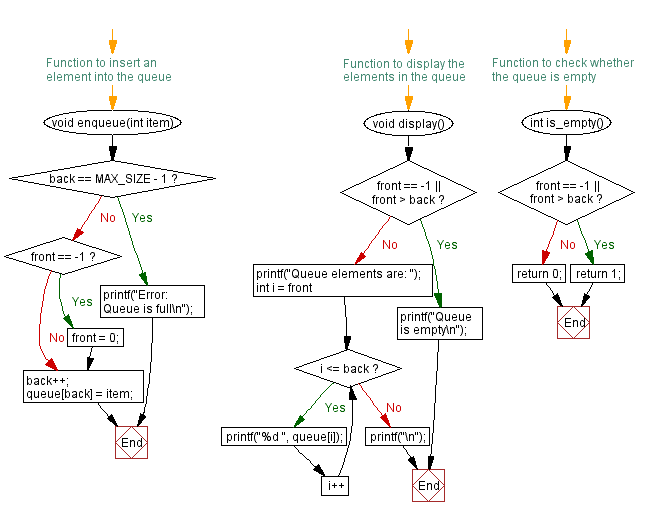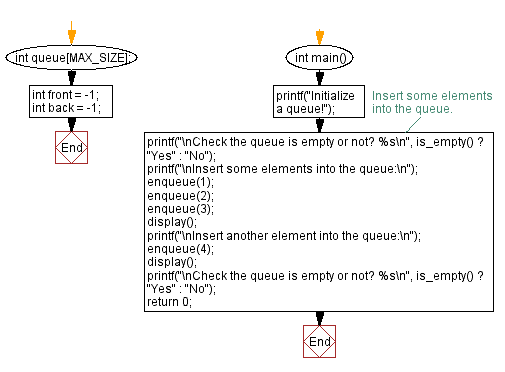﻿ C - Implement a queue using an array with insert, display

# C Exercises: Implement a queue using an array with insert, display

## C Queue: Exercise-1 with Solution

Write a C program to implement a queue using an array. Programs should contain functions for inserting elements into the queue, displaying queue elements, and checking whether the queue is empty or not.

Sample Solution:

C Code:

``````#include <stdio.h>

#define MAX_SIZE 100
int queue[MAX_SIZE];
int front = -1;
int back = -1;
// Function to insert an element into the queue
void enqueue(int item) {
if (back == MAX_SIZE - 1) {
printf("Error: Queue is full\n");
return;
}
if (front == -1) {
front = 0;
}
back++;
queue[back] = item;
}

// Function to display the elements in the queue
void display() {
if (front == -1 || front > back) {
printf("Queue is empty\n");
return;
}
printf("Queue elements are: ");
for (int i = front; i <= back; i++) {
printf("%d ", queue[i]);
}
printf("\n");
}
// Function to check whether the queue is empty
int is_empty() {
if (front == -1 || front > back) {
return 1;
}
return 0;
}
int main() {
printf("Initialize a queue!");
// Insert some elements into the queue.
printf("\nCheck the queue is empty or not? %s\n", is_empty() ? "Yes" : "No");
printf("\nInsert some elements into the queue:\n");
enqueue(1);
enqueue(2);
enqueue(3);
display();
printf("\nInsert another element into the queue:\n");
enqueue(4);
display();
printf("\nCheck the queue is empty or not? %s\n", is_empty() ? "Yes" : "No");
return 0;
}
```
```

Sample Output:

```Initialize a queue!
Check the queue is empty or not? Yes

Insert some elements into the queue:
Queue elements are: 1 2 3

Insert another element into the queue:
Queue elements are: 1 2 3 4
```

Flowchart:C Programming Code Editor:

Have another way to solve this solution? Contribute your code (and comments) through Disqus.

Previous: C Queue Exercises Home
Next: Remove an element from a queue.

What is the difficulty level of this exercise?

Test your Programming skills with w3resource's quiz.

﻿

## C Programming: Tips of the Day

C Programming - How do you pass a function as a parameter in C?

Declaration

A prototype for a function which takes a function parameter looks like the following:

```void func ( void (*f)(int) );
```

This states that the parameter f will be a pointer to a function which has a void return type and which takes a single int parameter. The following function (print) is an example of a function which could be passed to func as a parameter because it is the proper type:

```void print ( int x ) {
printf("%d\n", x);
}
```

Function Call

When calling a function with a function parameter, the value passed must be a pointer to a function. Use the function's name (without parentheses) for this:

```func(print);
```

would call func, passing the print function to it.

Function Body

As with any parameter, func can now use the parameter's name in the function body to access the value of the parameter. Let's say that func will apply the function it is passed to the numbers 0-4. Consider, first, what the loop would look like to call print directly:

```for ( int ctr = 0 ; ctr < 5 ; ctr++ ) {
print(ctr);
}
```

Since func's parameter declaration says that f is the name for a pointer to the desired function, we recall first that if f is a pointer then *f is the thing that f points to (i.e. the function print in this case). As a result, just replace every occurrence of print in the loop above with *f:

```void func ( void (*f)(int) ) {
for ( int ctr = 0 ; ctr < 5 ; ctr++ ) {
(*f)(ctr);
}
}
```

Ref : https://bit.ly/3skw9Um